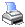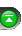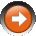﻿ Valid Input Data for Indicator Methods
 NinjaScript > Language Reference > Indicator Methods > Valid Input Data for Indicator MethodsIndicator method can accept the following valid forms of input data.

Default Input
Default input of the custom indicator, Market Analyzer column or strategy is used as default input if input is not specified.

 // Printing the current value of the 10 period SMA of closing prices // using the default input. double value = SMA(10); Print("The current SMA value is " + value.ToString());

Price Series

Open, High, Low, Close and Volume can all be used as input for an indicator method.

 // Passing in the a price series of High prices and printing out the current value of the // 14 period simple moving average double value = SMA(High, 14); Print("The current SMA value is " + value.ToString());

Indicator
Indicators can be used as input for indicators.

 // Printing the current value of the 20 period simple moving average of a 14 period RSI // using a data series of closing prices double value = SMA(RSI(Close, 14), 20); Print("The current SMA value is " + value.ToString());

DataSeries
DataSeries can be used as input for indicators.

 // Instantiating a new DataSeries object and passing it in as input to calculate // a simple moving average DataSeries myDataSeries = new DataSeries(this); double value = SMA(myDataSeries, 20);

Bars Object
A Bars object (holds a series of bar object that contain OHLC data) can be used as input for indicators.

 // Passing in the second Bars object held in a multi-instrument and timeframe strategy // The default value used for the SMA calculation is the close price double value = SMA(BarsArray, 20); Print("The current SMA value is " + value.ToString());

Tips

1. The input series of an indicator cannot be the hosting indicator as it will cause recursive loops.

 // Using the hosting indicator like below will cause errors with recursive loops double value = SMA(this, 20);